# How to Convert Lowercase to Proper or Sentence Case in Excel?

Sometimes in Excel, you may have observed that the sentences in the sheet are not in proper format. If we can make our data look ugly, Manually resolving this issue can be a time-consuming and lengthy process. We can solve this process in a faster and more accurate way using the formulas supported by Excel.

This tutorial will help you understand how lowercase letters can be converted to proper case or sentence case in Excel. If only the first letter of a sentence is capital, then it is called sentence case, and if the first letters of all words of a sentence are upper case, then they are known as proper case.

## Converting Lowercase to Proper Case in Excel

Here we will get any one of the results using the PROPER formula, then get all the results using the auto-fill handle. Let's look at a simple procedure for converting lowercase to proper case in Excel.

### Step 1

Let us consider an excel sheet where the data is a list of sentences in lowercase, similar to the below image.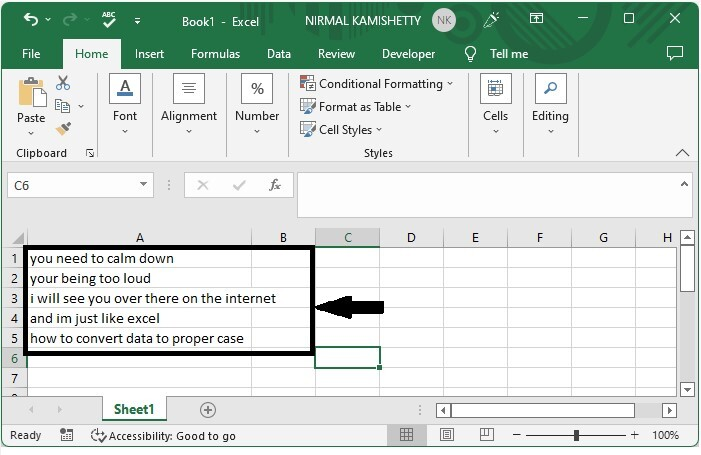Now click on the empty cell in our case, cell B1, and enter the formula as =PROPER(A1) and click enter to get our first result. In the formula, A1 is the address of the sentence.

Empty cell > formula > enter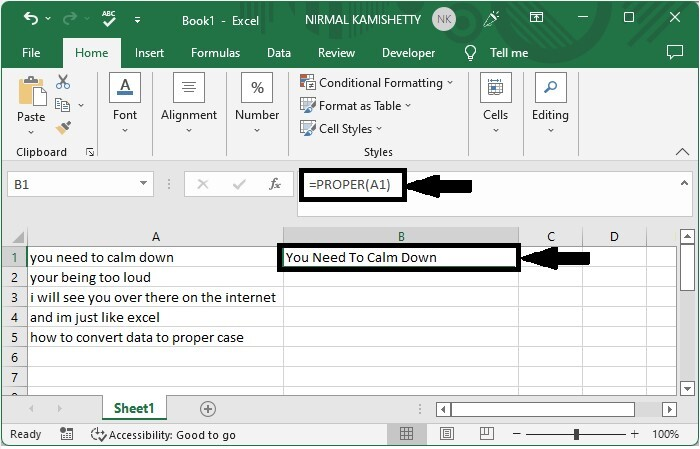### Step 2

Then drag down from the first result using the auto-fill handle to get all the results that look similar to the below image.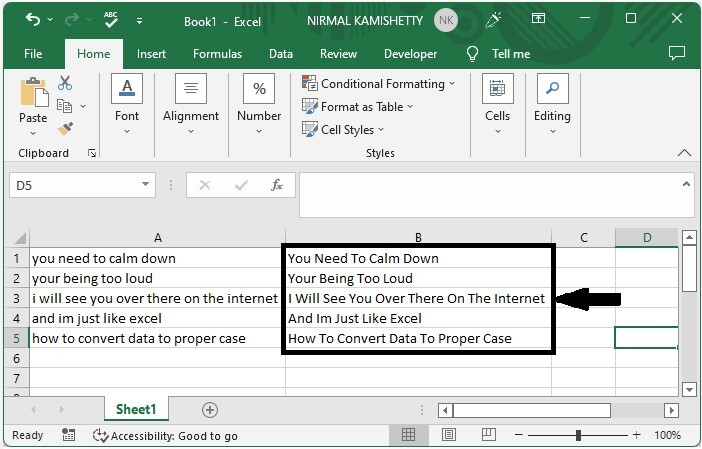## Converting Lowercase to Sentence Case in Excel

Here we will get any one result using the SUBSTITUTE formula, then get all the results using the auto-fill handle. Let's take a look at a simple procedure for converting lowercase to sentence case in Excel.

### Step 1

Let us consider the same data that we used in the above example.

Then, in our case, cell B1, click on an empty cell and enter the formula as follows −

=SUBSTITUTE(LOWER(A1),CHAR(CODE(A1)),UPPER(CHAR(CODE(A1))),1) and click Enter to get our first value.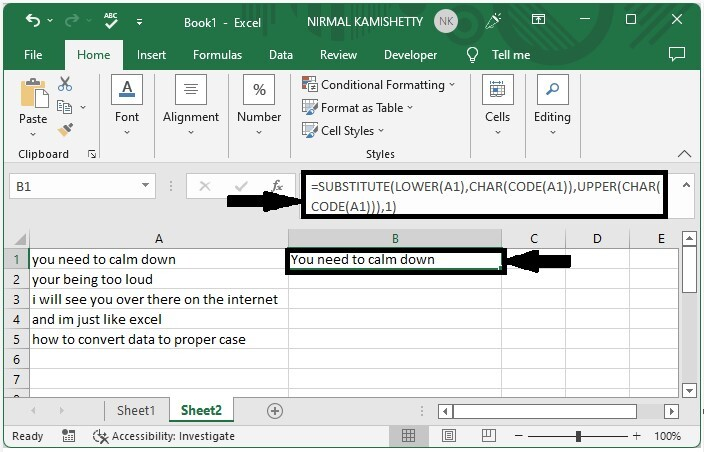### Step 2

Then drill down from the first result using the auto-fill handle to get all the results that look similar to the below image.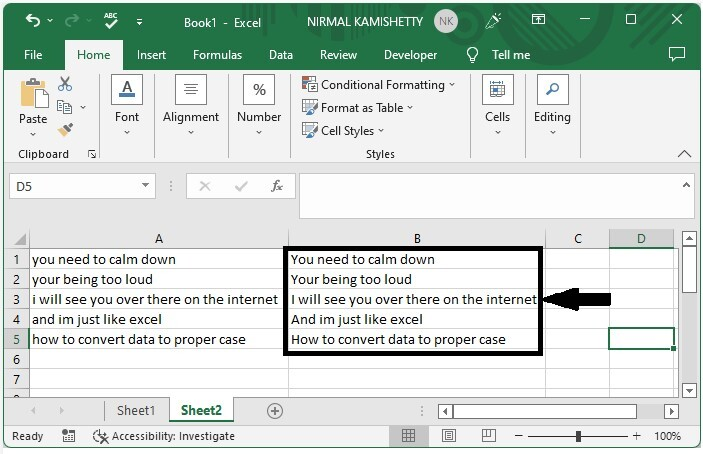## Conclusion

In this tutorial, we used a simple example to demonstrate how we can convert lowercase to proper case and sentence case in Excel.

Updated on: 24-Feb-2023

448 Views